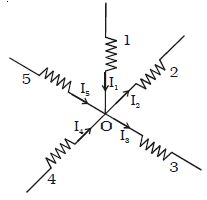# Kirchoff’s Current Law

Kirchoff’s current law states that the algebraic sum of the currents meeting at any junction in a circuit is zero.

The convention is that, the current flowing towards a junction is positive and the current flowing away from the junction is negative. Let 1,2,3,4 and 5 be the conductors meeting at a junction O in an electrical circuit (Figure).Fig: Kirchoff’s current law

Let I1, I2, I3, I4 and I5 be the currents passing through the conductors respectively. According to Kirchoff’s first law.

I1 + (−I2) + (−I3) + I4 + I5 = 0 or I1 + I4 + I5 = I2 + I3.

The sum of the currents entering the junction is equal to the sum of the currents leaving the junction. This law is a consequence of conservation of charges.